# Build an Artificial Neural Network From Scratch: Part 2

The second article in this series focuses on building an Artificial Neural Network using the Numpy Python library.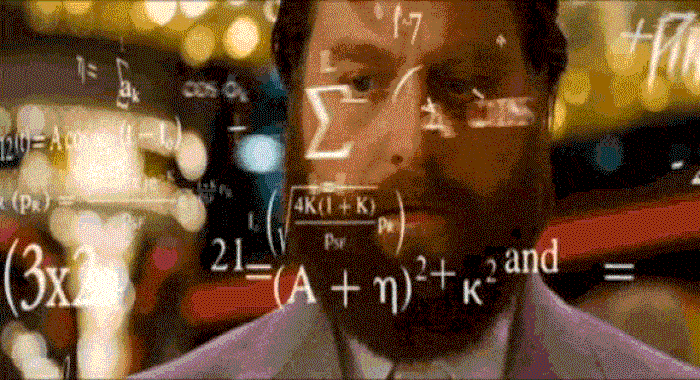In my previous article, Build an Artificial Neural Network(ANN) from scratch: Part-1 we started our discussion about what are artificial neural networks; we saw how to create a simple neural network with one input and one output layer, from scratch in Python. Such a neural network is called a perceptron. However, real-world neural networks, capable of performing complex tasks such as image classification and stock market analysis, contain multiple hidden layers in addition to the input and output layer.

In the previous article, we concluded that a Perceptron is capable of finding a linear decision boundary. We used the perceptron to predict whether a person is diabetic or not using a dummy dataset. However, a perceptron is not capable of finding non-linear decision boundaries.

In this article, we will develop a neural network with one input layer, one hidden layer, and one output layer. We will see that the neural network that we will develop will be capable of finding non-linear boundaries.

### Generating a dataset

Let’s start by generating a dataset we can play with. Fortunately, scikit-learn has some useful dataset generators, so we don’t need to write the code ourselves. We will go with the make_moons function.

``````from sklearn import datasets
np.random.seed(0)
feature_set, labels = datasets.make_moons(300, noise=0.20)
plt.figure(figsize=(10,7))
plt.scatter(feature_set[:,0], feature_set[:,1], c=labels, cmap=plt.cm.Spectral)``````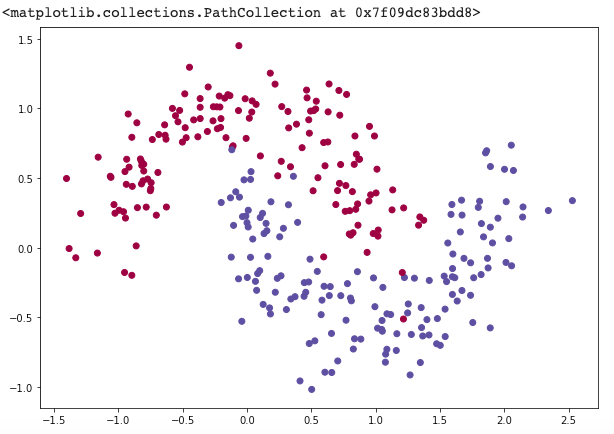The dataset we generated has two classes, plotted as red and blue points. You can think of the blue dots as male patients and the red dots as female patients, with the x-axis and y-axis being medical measurements.

Our goal is to train a Machine Learning classifier that predicts the correct class (male or female) given the `x` and `y`coordinates. Note that the data is not linearly separable, we can’t draw a straight line that separates the two classes. This means that linear classifiers, such as ANN without any hidden layer or even Logistic Regression, won’t be able to fit the data unless you hand-engineer non-linear features (such as polynomials) that work well for the given dataset.

### Neural Networks with One Hidden Layer

Here’s our simple network: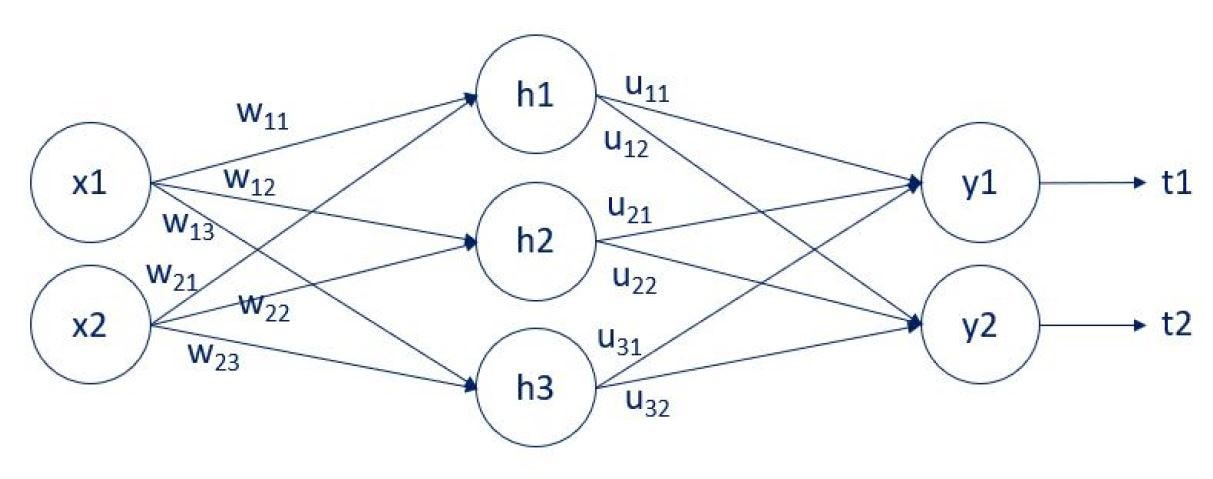We have two inputs: x1 and x2. There is a single hidden layer with 3 units (nodes): h1, h2, and h3. Finally, there are two outputs: y1 and y2. The arrows that connect them are the weights. There are two weights matrices: w, and u. The w weights connect the input layer and the hidden layer. The u weights connect the hidden layer and the output layer. We have employed the letters w, and u, so it is easier to follow the computation to follow. You can also see that we compare the outputs y1 and y2 with the targets t1 and t2.

There is one last letter we need to introduce before we can get to the computations. Let a be the linear combination prior to activation. Thus, we have: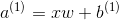and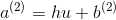Since we cannot exhaust all activation functions and all loss functions, we will focus on two of the most common. A sigmoid activation and an L2-norm loss. With this new information and the new notation, the output y is equal to the activated linear combination.

Therefore, for the output layer, we have: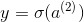while for the hidden layer: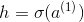We will examine backpropagation for the output layer and the hidden layer separately, as the methodologies differ.

I would like to remind you that: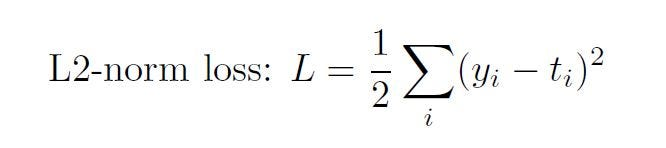The sigmoid function is: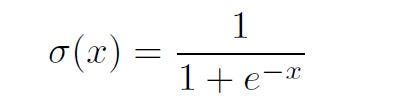and its derivative is: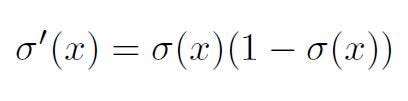### Backpropagation for the output layer

In order to obtain the update rule: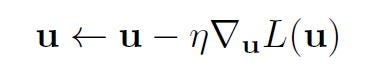we must calculate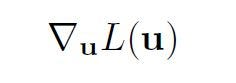Let’s take a single weight uij . The partial derivative of the loss w.r.t. uij equals: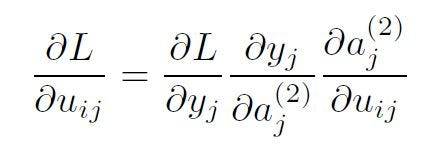where i corresponds to the previous layer (input layer for this transformation) and j corresponds to the next layer (output layer of the transformation). The partial derivatives were computed simply following the chain rule.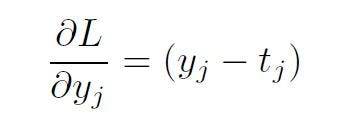following the L2-norm loss derivative.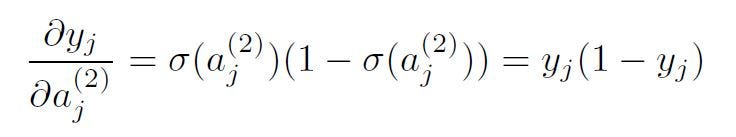following the sigmoid derivative.

Finally, the third partial derivative is simply the derivative of:So,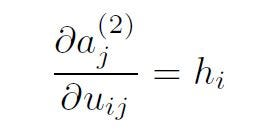Replacing the partial derivatives in the expression above, we get: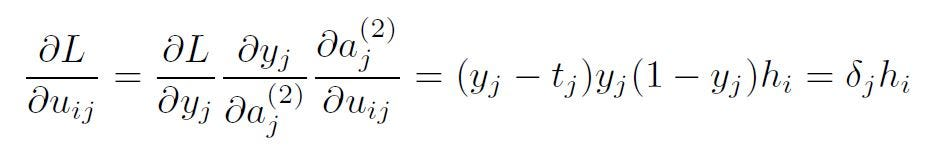Therefore, the update rule for a single weight for the output layer is given by: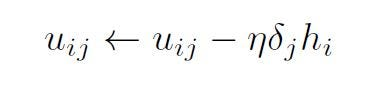### 5. Backpropagation of a hidden layer

Similarly to the backpropagation of the output layer, the update rule for a single weight, wij would depend on: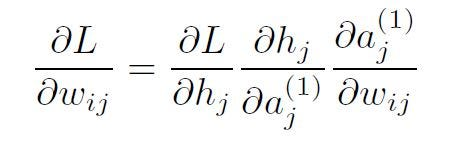following the chain rule. Taking advantage of the results we have so far for transformation using the sigmoid activation and the linear model, we get: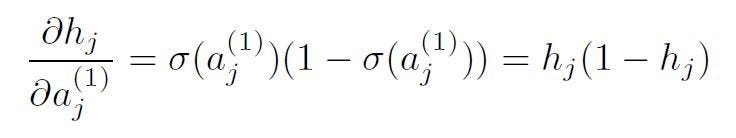and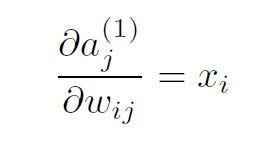The actual problem for backpropagation comes from the term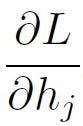That’s due to the fact that there is no “hidden” target. You can follow the solution for weight w11 below. It is advisable to keep a look on the NN diagram shown above, while going through the computations.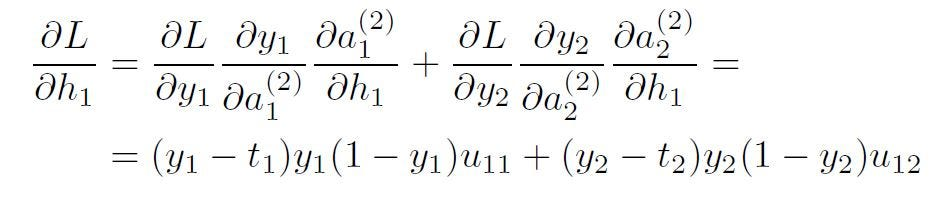From here, we can calculate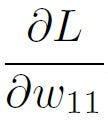Which was what we wanted. The final expression is: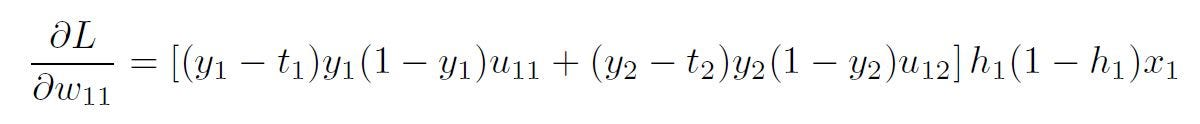The generalized form of this equation is: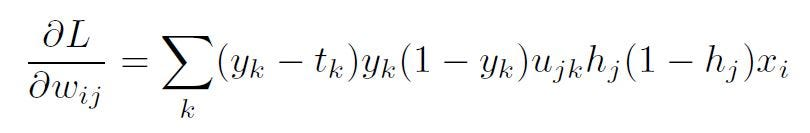### Backpropagation generalization

Using the results for backpropagation for the output layer and the hidden layer, we can put them together in one formula, summarizing backpropagation, in the presence of L2-norm loss and sigmoid activations.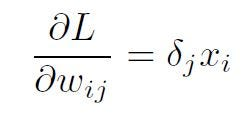where for a hidden layer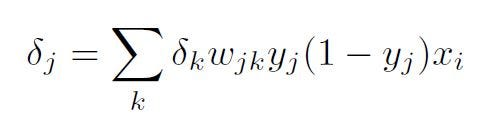### Code for Neural Networks with One Hidden Layer

Now let’s implement the neural network that we just discussed in Python from scratch. We will again try to classify the non-linear data that we created above.

We start by defining some useful variables and parameters for gradient descent like training dataset size, dimensions of input and output layers.

``````num_examples = len(X) # training set size
nn_input_dim = 2 # input layer dimensionality
nn_output_dim = 2 # output layer dimensionality``````

Also defining the Gradient descent parameters.

``````epsilon = 0.01 # learning rate for gradient descent
reg_lambda = 0.01 # regularization strength``````

First, let’s implement the loss function we defined above. We use this to evaluate how well our model is doing:

``````# Helper function to evaluate the total loss on the dataset
def calculate_loss(model, X, y):
num_examples = len(X)  # training set size
W1, b1, W2, b2 = model['W1'], model['b1'], model['W2'], model['b2']
# Forward propagation to calculate our predictions
z1 = X.dot(W1) + b1
a1 = np.tanh(z1)
z2 = a1.dot(W2) + b2
exp_scores = np.exp(z2)
probs = exp_scores / np.sum(exp_scores, axis=1, keepdims=True)
# Calculating the loss
corect_logprobs = -np.log(probs[range(num_examples), y])
data_loss = np.sum(corect_logprobs)
# Add regulatization term to loss (optional)
data_loss += Config.reg_lambda / 2 * (np.sum(np.square(W1)) + np.sum(np.square(W2)))
return 1. / num_examples * data_loss``````

We also implement a helper function to calculate the output of the network. It does forward propagation and returns the class with the highest probability.

``````def predict(model, x):
W1, b1, W2, b2 = model['W1'], model['b1'], model['W2'], model['b2']
# Forward propagation
z1 = x.dot(W1) + b1
a1 = np.tanh(z1)
z2 = a1.dot(W2) + b2
exp_scores = np.exp(z2)
probs = exp_scores / np.sum(exp_scores, axis=1, keepdims=True)
return np.argmax(probs, axis=1)``````

Finally, here comes the function to train our Neural Network. It implements batch gradient descent using the backpropagation derivates we found above.

This function learns parameters for the neural network and returns the model.

nn_hdim: Number of nodes in the hidden layer
num_passes: Number of passes through the training data for gradient descent
print_loss: If True, print the loss every 1000 iterations

``````def build_model(X, y, nn_hdim, num_passes=20000, print_loss=False):
# Initialize the parameters to random values. We need to learn these.
num_examples = len(X)
np.random.seed(0)
W1 = np.random.randn(Config.nn_input_dim, nn_hdim) / np.sqrt(Config.nn_input_dim)
b1 = np.zeros((1, nn_hdim))
W2 = np.random.randn(nn_hdim, Config.nn_output_dim) / np.sqrt(nn_hdim)
b2 = np.zeros((1, Config.nn_output_dim))# This is what we return at the end
model = {}# Gradient descent. For each batch...
for i in range(0, num_passes):# Forward propagation
z1 = X.dot(W1) + b1
a1 = np.tanh(z1)
z2 = a1.dot(W2) + b2
exp_scores = np.exp(z2)
probs = exp_scores / np.sum(exp_scores, axis=1, keepdims=True)# Backpropagation
delta3 = probs
delta3[range(num_examples), y] -= 1
dW2 = (a1.T).dot(delta3)
db2 = np.sum(delta3, axis=0, keepdims=True)
delta2 = delta3.dot(W2.T) * (1 - np.power(a1, 2))
dW1 = np.dot(X.T, delta2)
db1 = np.sum(delta2, axis=0)# Add regularization terms (b1 and b2 don't have regularization terms)
dW2 += Config.reg_lambda * W2
dW1 += Config.reg_lambda * W1# Gradient descent parameter update
W1 += -Config.epsilon * dW1
b1 += -Config.epsilon * db1
W2 += -Config.epsilon * dW2
b2 += -Config.epsilon * db2# Assign new parameters to the model
model = {'W1': W1, 'b1': b1, 'W2': W2, 'b2': b2}# Optionally print the loss.
# This is expensive because it uses the whole dataset, so we don't want to do it too often.
if print_loss and i % 1000 == 0:
print("Loss after iteration %i: %f" % (i, calculate_loss(model, X, y)))return model``````

Finally the main method:

``````def main():
X, y = generate_data()
model = build_model(X, y, 3, print_loss=True)
visualize(X, y, model)``````

Print loss after every 1000 iterations: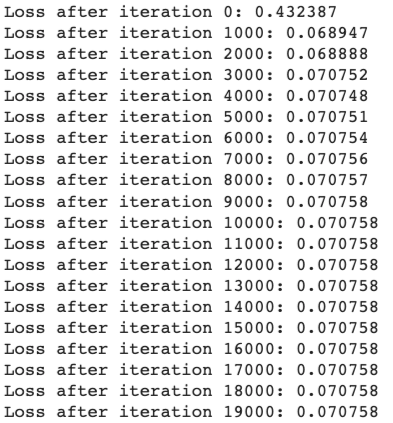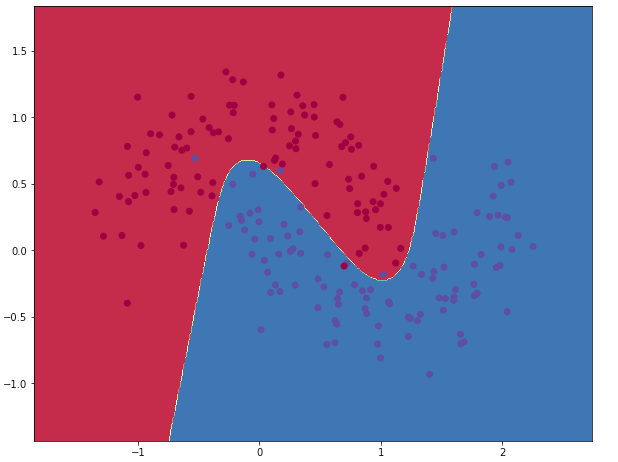Classification when the number of nodes in the hidden layer is 3

Now Let’s now get a sense of how varying the hidden layer size affects the result.

``````hidden_layer_dimensions = [1, 2, 3, 4, 5, 20, 50]
for i, nn_hdim in enumerate(hidden_layer_dimensions):
plt.subplot(5, 2, i+1)
plt.title('Hidden Layer size %d' % nn_hdim)
model = build_model(X, y,nn_hdim, 20000, print_loss=False)
plot_decision_boundary(lambda x:predict(model,x), X, y)
plt.show()``````We can see that a hidden layer of low dimensionality nicely captures the general trend of our data. Higher dimensionalities are prone to overfitting. They are “memorizing” the data as opposed to fitting the general shape.

If we were to evaluate our model on a separate test set the model with a smaller hidden layer size would likely perform better due to better generalization. We could counteract overfitting with stronger regularization, but picking the correct size for the hidden layer is a much more “economical” solution.

You can get the whole code in this GitHub repo.

nageshsinghc4/Artificial-Neural-Network-from-scratch-python

### Conclusion

So in this article, we saw how we can mathematically derive a neural network with one hidden layer and we also created a neural network with 1 hidden layer, from scratch in Python using NumPy.

Well, this concludes the two-article series on building an Artificial Neural Network from scratchI hope you guys have enjoyed reading it, feel free to share your comments/thoughts/feedback in the comment section.

Bio: Nagesh Singh Chauhan is a Big data developer at CirrusLabs. He has over 4 years of working experience in various sectors like Telecom, Analytics, Sales, Data Science having specialisation in various Big data components.

Original. Reposted with permission.

Related: